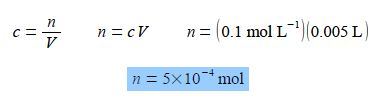Mathematics

# Mathematical Example: Concentration of Molecules in Solutions

Chemists routinely need to know and alter the concentrations of solutions in the lab. The concentration of a solute is c mol L-1 and is given in terms of the number of moles of the solute, n mol, and the volume of the solution, V, measured in litres, symbol L. The SI unit of volume is the metre cubed m3 which is 1000 L, however, chemists often use the sub-multiple unit litre as it is more convenient than the cubic metre. The litre, L, is identical to the decimetre cubed, dm3.

c = n/V

If 5 mL of a 0.1 mol L-1 aqueous solution is diluted with 10 mL of water, calculate the concentration of the new solution. Note that mL is the correct symbol for millilitre or one thousandth of a litre L (rather than the old-fashioned symbol ml which is still commonly seen).

Solution

The number of moles of the solute remains constant when the concentration is altered by diluting the solution with more solvent, n is constant as c varies Rearranging the equation so that the unknown constant quantity n becomes the subject of the equation and then substituting in the original physical quantities,It is usually less confusing to carry out calculations in the base unit, here the L, rather than multiples or sub-multiples such as the mL.